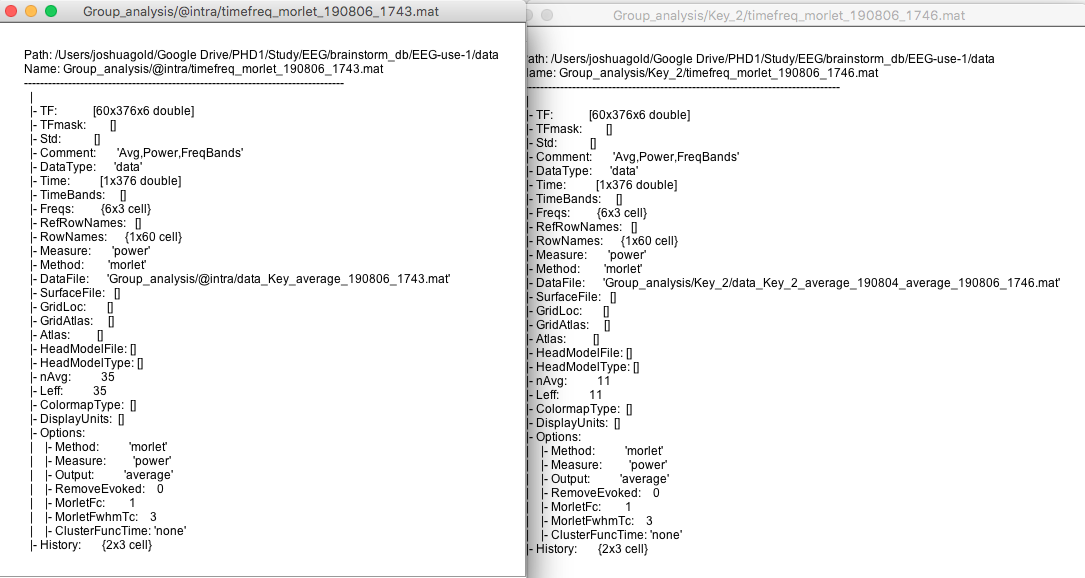# Statistics: Timefreq parametric test error "Files A and B do not have the same number of signals or time samples."

Hello
I am trying to run a paired parametric test on mortlet time freq analyses i have run.
My process has been to average the participants multiple trials and then average them. I then ran a mortletts on the average.
I then took these time freqs and placed them in the corresponding process A and B boxes.
after running the analysis i receive an error that states
"Files A and B do not have the same number of signals or time samples."
My epochs are 1500ms but I have also selected smaller time periods of 100ms. My channel numbers and frequency sampling is the same. the only difference between files that i have found is the number of files averaged.
I am unsure how to move beyond this error. Any help would be appreciated.
Many thanks.
Josh

My process has been to average the participants multiple trials and then average them. I then ran a mortletts on the average.

You should compute the time-frequency decomposition of each single trial and average in power, as explained in the tutorial:
https://neuroimage.usc.edu/brainstorm/Tutorials/TimeFrequency#MEG_recordings:_Single_trials

I then took these time freqs and placed them in the corresponding process A and B boxes.
after running the analysis i receive an error that states
"Files A and B do not have the same number of signals or time samples."

There are pairs of files (A/B) that do not match in terms of data dimension. You can check the dimensions [Nsignals x Ntime x Nfreq] of the TF matrix by right-clicking on a file > File > View file contents. You need to fix the pipeline you used to create these files (either by normalizing the number of channels, or the number of time samples, or the number of frequency bins). Some processes in the category "Standardize" may help you.

Thank you for your response Francois. I will use this method you offered. Is this the best option for repeated measures?
Also I have checked the TF matrix and they are identical (attached) the only difference was the number of trials used in the average. Therefore it make me wonder what i am meant to standardize if the TF matrix is the same.
I appreciate any thoughts you may have.
Thank you
JoshRead the articles referenced in the time-frequency tutorial, they explain the notions of evoked vs. induced brain activity. You can extract the second one (not time-locked) only by computing the time-frequency power on each trial, and averaging these power values.

Also I have checked the TF matrix and they are identical (attached) the only difference was the number of trials used in the average. Therefore it make me wonder what i am meant to standardize if the TF matrix is the same.

Have you checked all the files for all the subjects?
Have you tried with or without the option "Match signals between files using their names"? If the order or names of the signals in the different TF files in input are different, the results can be random... Check the field "RowNames" in the different files (export the files to Matlab and compare the signal names if you are not sure)